#### Stepping along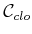The RDT algorithm given Figure 5.21 can be applied directly; however, the STOPPING-CONFIGURATION function in line 4 must be changed to account for both obstacles and the constraints that define. Figure 7.22 shows one general approach that is based on numerical continuation . An alternative is to use inverse kinematics, which is part of the approach described in Section 7.4.2. The nearest RDT vertex,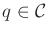, to the sample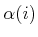is first computed. Let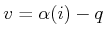, which indicates the direction in which an edge would be made from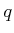if there were no constraints. A local motion is then computed by projecting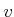into the tangent plane7.3 ofat the point. Sinceis generally nonlinear, the local motion produces a point that is not precisely on. Some numerical tolerance is generally accepted, and a small enough step is taken to ensure that the tolerance is maintained. The process iterates by computingwith respect to the new point and moving in the direction ofprojected into the new tangent plane. If the error threshold is surpassed, then motions must be executed in the normal direction to return to. This process of executing tangent and normal motions terminates when progress can no longer be made, due either to the alignment of the tangent plane (nearly perpendicular to) or to an obstacle. This finally yields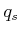, the stopping configuration. The new path followed in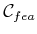is no longer a straight line'' as was possible for some problems in Section 5.5; therefore, the approximate methods in Section 5.5.2 should be used to create intermediate vertices along the path.

In each iteration, the tangent plane computation is computed at some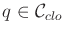as follows. The differential configuration vector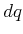lies in the tangent space of a constraint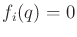if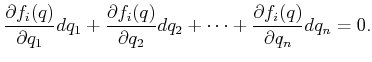(7.22)

This leads to the following homogeneous system for all of thepolynomials in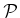that define the closure constraints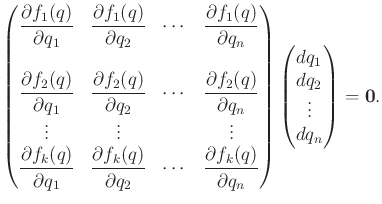(7.23)

If the rank of the matrix is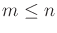, then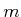configuration displacements can be chosen independently, and the remaining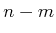parameters must satisfy (7.23). This can be solved using linear algebra techniques, such as singular value decomposition (SVD) [399,961], to compute an orthonormal basis for the tangent space at. Let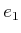,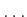,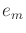, denote these-dimensional basis vectors. The components of the motion direction are obtained from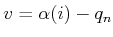. First, construct the inner products,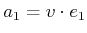,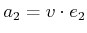,,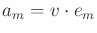. Using these, the projection ofin the tangent plane is the-dimensional vector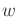given by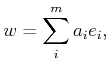(7.24)

which is used as the direction of motion. The magnitude must be appropriately scaled to take sufficiently small steps. Sinceis generally curved, a linear motion leaves. A motion in the inward normal direction is then required to move back onto.

Since the dimensionofis less than, the procedure just described can only produce numerical approximations to paths in. This problem also arises in implicit curve tracing in graphics and geometric modeling . Therefore, each constraint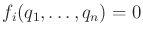is actually slightly weakened to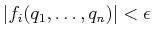for some fixed tolerance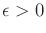. This essentially thickens''so that its dimension is. As an alternative to computing the tangent plane, motion directions can be sampled directly inside of this thickened region without computing tangent planes. This results in an easier implementation, but it is less efficient .

Steven M LaValle 2020-08-14